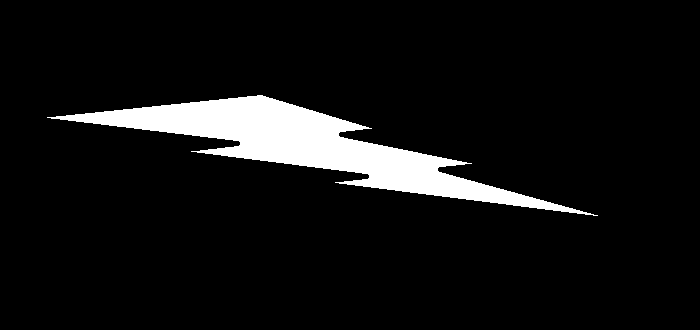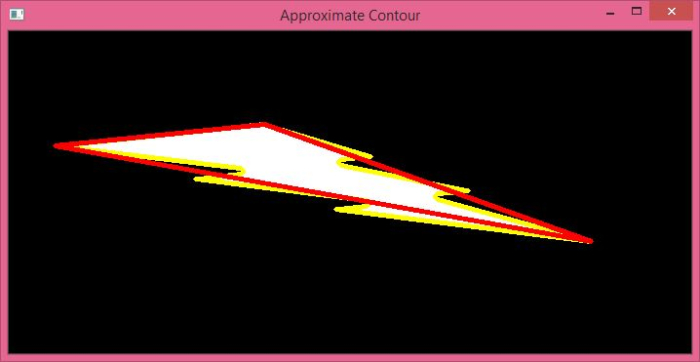# How to approximate a contour shape in an image using OpenCV Python?

The function cv2.approxPolyDP() approximates a contour shape to another shape with less number of vertices. It accepts the following arguments −

• cnt − The array of the contour points.

• epsilon − Maximum distance from contour to approximated contour. A wise selection of epsilon is needed to get the correct output.

## Syntax

The following syntax are used to approximate a contour shape

epsilon = 0.01*cv2.arcLength(cnt,True)
approx = cv2.approxPolyDP(cnt,epsilon,True)


## Steps

You can use the following steps to approximate a contour shape in an image −

Import the required library. In all the following Python examples, the required Python library is OpenCV. Make sure you have already installed it.

import cv2


img = cv2.imread('approx1.png')
gray = cv2.cvtColor(img, cv2.COLOR_BGR2GRAY)


Apply thresholding on the grayscale image to create a binary image. Adjust the second parameter to get a better contour detection.

ret,thresh = cv2.threshold(gray,150,255,0)


Find the contours in the image using cv2.findContours() function.

contours, _ = cv2.findContours(thresh, cv2.RETR_TREE, cv2.CHAIN_APPROX_SIMPLE)


Select a contour (say first contour) cnt from the lists of contours.

cnt = contours


Define the precision, the epsilon. This step is very important. The correct approximation of the contour depends on selection of epsilon.

epsilon = 0.01*cv2.arcLength(cnt,True)


Compute the approximate contour points using cv2.approxPolyDP() function.

approx = cv2.approxPolyDP(cnt,epsilon,True)


Draw both contours and approximate contours on the input image with different colors.

cv2.drawContours(img, [cnt], -1, (0,255,255), 3)
cv2.drawContours(img, [approx], -1, (0,0,255), 3)


Display the image with drawn contours and approximate contours on it.

cv2.imshow("Approximate Contour", img)
cv2.waitKey(0)
cv2.destroyAllWindows()


## Example

In the Python code below, we find the approximate contour of an object in the input image. We also draw the approximate contour on the input image.

import cv2
import numpy as np

# convert the image to grayscale
gray = cv2.cvtColor(img, cv2.COLOR_BGR2GRAY)

# apply thresholding to convert the grayscale image to a binary image
ret,thresh = cv2.threshold(gray,150,255,0)

# find the contours
contours,hierarchy = cv2.findContours(thresh, cv2.RETR_TREE,cv2.CHAIN_APPROX_SIMPLE)
print("Number of contours detected:",len(contours))

# take first contour
cnt = contours

# define the precision
epsilon = 0.01*cv2.arcLength(cnt,True)

# approximate the contour
approx = cv2.approxPolyDP(cnt,epsilon,True)

# draw the contour on the input image
cv2.drawContours(img, [cnt], -1, (0,255,255), 3)

# draw the approximate contour on the input image
cv2.drawContours(img, [approx], -1, (0,0,255), 3)

# display the image with drawn contours and approximate contours
cv2.imshow("Approximate Contour", img)
cv2.waitKey(0)
cv2.destroyAllWindows()


We will use the following image as the Input File in the above program code.## Output

When you execute the above code, it will produce the following output.

Number of contours detected: 1


And we get the following window, showing the outputIn the above output image, the contour of the object is shown in yellow color and the approximate contour of the object is shown in red color. Notice the difference between the two contours.